# KR20120126572A - Cognitive radio base station and communication method of the cognitive radio base station in a multi-user multiple-input multiple output cognitive radio network system - Google Patents

Cognitive radio base station and communication method of the cognitive radio base station in a multi-user multiple-input multiple output cognitive radio network system Download PDF

## Info

Publication number
KR20120126572A
KR20120126572A KR1020110044463A KR20110044463A KR20120126572A KR 20120126572 A KR20120126572 A KR 20120126572A KR 1020110044463 A KR1020110044463 A KR 1020110044463A KR 20110044463 A KR20110044463 A KR 20110044463A KR 20120126572 A KR20120126572 A KR 20120126572A
Authority
KR
South Korea
Prior art keywords
cognitive radio
matrix
base station
weight vector
beamforming weight
Prior art date
Application number
KR1020110044463A
Other languages
Korean (ko)
Inventor
박재현
엄중선
정병장
정회윤
황성현
Original Assignee
한국전자통신연구원
Priority date (The priority date is an assumption and is not a legal conclusion. Google has not performed a legal analysis and makes no representation as to the accuracy of the date listed.)
Filing date
Publication date
Application filed by 한국전자통신연구원 filed Critical 한국전자통신연구원
Priority to KR1020110044463A priority Critical patent/KR20120126572A/en
Publication of KR20120126572A publication Critical patent/KR20120126572A/en

## Links

• 230000001149 cognitive Effects 0.000 title claims abstract description 305
• 238000004891 communication Methods 0.000 title claims abstract description 123
• 239000011159 matrix materials Substances 0.000 claims abstract description 221
• 238000000034 methods Methods 0.000 claims abstract description 23
• 238000000354 decomposition reactions Methods 0.000 claims description 23
• 230000000875 corresponding Effects 0.000 claims description 15
• 238000004364 calculation methods Methods 0.000 claims description 9
• 238000007493 shaping process Methods 0.000 claims 1
• 238000004422 calculation algorithm Methods 0.000 description 14
• 238000010586 diagram Methods 0.000 description 12
• 230000005540 biological transmission Effects 0.000 description 9
• 238000005457 optimization Methods 0.000 description 4
• 230000015572 biosynthetic process Effects 0.000 description 2
• 238000005755 formation reaction Methods 0.000 description 2
• 238000007781 pre-processing Methods 0.000 description 2
• 238000009795 derivation Methods 0.000 description 1
• 238000005516 engineering process Methods 0.000 description 1
• 238000005562 fading Methods 0.000 description 1
• 230000004048 modification Effects 0.000 description 1
• 238000006011 modification reaction Methods 0.000 description 1
• 230000003287 optical Effects 0.000 description 1
• 229910052709 silver Inorganic materials 0.000 description 1
• 239000004332 silver Substances 0.000 description 1

## Images

•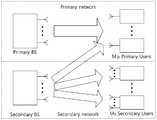•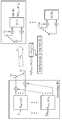••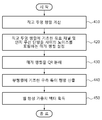••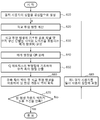••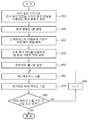•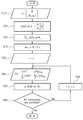•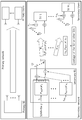•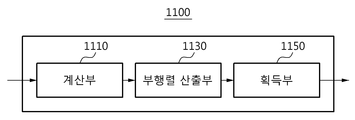•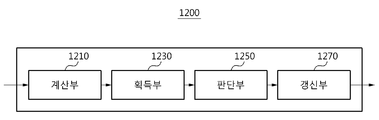•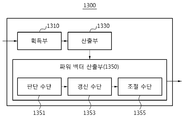## Classifications

• HELECTRICITY
• H04ELECTRIC COMMUNICATION TECHNIQUE
• H04BTRANSMISSION
• H04B7/00Radio transmission systems, i.e. using radiation field
• H04B7/02Diversity systems; Multi-antenna system, i.e. transmission or reception using multiple antennas
• H04B7/04Diversity systems; Multi-antenna system, i.e. transmission or reception using multiple antennas using two or more spaced independent antennas
• H04B7/0413MIMO systems
• H04B7/0426Power distribution
• H04B7/043Power distribution using best eigenmode, e.g. beam forming or beam steering
• HELECTRICITY
• H04ELECTRIC COMMUNICATION TECHNIQUE
• H04BTRANSMISSION
• H04B7/00Radio transmission systems, i.e. using radiation field
• H04B7/02Diversity systems; Multi-antenna system, i.e. transmission or reception using multiple antennas
• H04B7/04Diversity systems; Multi-antenna system, i.e. transmission or reception using multiple antennas using two or more spaced independent antennas
• H04B7/0413MIMO systems
• H04B7/0452Multi-user MIMO systems
• HELECTRICITY
• H04ELECTRIC COMMUNICATION TECHNIQUE
• H04BTRANSMISSION
• H04B7/00Radio transmission systems, i.e. using radiation field
• H04B7/02Diversity systems; Multi-antenna system, i.e. transmission or reception using multiple antennas
• H04B7/04Diversity systems; Multi-antenna system, i.e. transmission or reception using multiple antennas using two or more spaced independent antennas
• H04B7/06Diversity systems; Multi-antenna system, i.e. transmission or reception using multiple antennas using two or more spaced independent antennas at the transmitting station
• H04B7/0613Diversity systems; Multi-antenna system, i.e. transmission or reception using multiple antennas using two or more spaced independent antennas at the transmitting station using simultaneous transmission
• H04B7/0615Diversity systems; Multi-antenna system, i.e. transmission or reception using multiple antennas using two or more spaced independent antennas at the transmitting station using simultaneous transmission of weighted versions of same signal
• H04B7/0617Diversity systems; Multi-antenna system, i.e. transmission or reception using multiple antennas using two or more spaced independent antennas at the transmitting station using simultaneous transmission of weighted versions of same signal for beam forming
• HELECTRICITY
• H04ELECTRIC COMMUNICATION TECHNIQUE
• H04WWIRELESS COMMUNICATION NETWORKS
• H04W16/00Network planning, e.g. coverage or traffic planning tools; Network deployment, e.g. resource partitioning or cells structures
• H04W16/14Spectrum sharing arrangements between different networks

## Abstract

A communication method of a cognitive radio base station in a multi-user multi-input / output cognitive radio system according to an embodiment of the present invention is directed to a communication method of a cognitive radio base station in a multi-user multi-input / output cognitive radio communication system including a cognitive radio base station and cognitive radio terminals. A method comprising: calculating an orthogonal projection matrix used to remove interference in a primary user from an interference channel using a zero forcing processing technique; And obtaining a beamforming weight vector for each of the cognitive radio terminals using the orthogonal projection matrix.

## Description

COGNITIVE RADIO BASE STATION AND COMMUNICATION METHOD OF THE COGNITIVE RADIO BASE STATION IN A MULTI-USER MULTIPLE-INPUT MULTIPLE OUTPUT COGNITIVE RADIO NETWORK SYSTEM}
The following embodiments are directed to a communication method of a cognitive radio base station and a cognitive radio base station in a multi-user multiple-input multiple-output (MIMO) cognitive radio (CR) communication system.
Cognitive radio (CR) communication technology is used to increase the number of data communication services, and when the available primary frequency is saturated, the existing primary communication system licensed for a specific frequency band does not use the band. Primary) Allows an unauthorized secondary communication system to use a particular frequency band under conditions that do not interfere with the communication of the communication system.
In a cognitive radio (CR) communication environment, it is necessary to utilize spatial freedom using multiple antennas in order to more efficiently use limited available frequencies, increase data rates, and not interfere with communication of a primary communication system.
To this end, a multiple-input multiple-output (MIMO) based beam-forming technique using multiple antennas in a cognitive radio base station and cognitive radio terminals is considered.
However, in a beamforming technique in which a cognitive radio terminal does not interfere with a primary communication system and maximizes a minimum received signal to interference and noise ratio (SINR) of a terminal, beamforming weights are obtained. This requires an optimization process that requires very complex iterations. Accordingly, there is a need for a method of obtaining a beamforming weight vector capable of increasing the channel capacity of a cognitive radio system with a lower complexity than the beamforming technique of maximizing the minimum SINR, and a related power control method.
One embodiment of the present invention provides beamforming with low computational complexity for improving channel capacity in a multi-user multi-input multiple-output (MIMO) cognitive radio (CR) communication system. A communication method of a cognitive radio base station and a cognitive radio base station capable of obtaining a weight vector is provided.
One embodiment of the present invention is a cognitive radio base station and a cognitive radio base station in a multi-user multi-input / output cognitive radio communication system that performs preprocessing and power control to not interfere with a primary user in a cognitive radio communication system. Provides a communication method.
A communication method of a cognitive radio base station in a multi-user multi-input / output cognitive radio system according to an embodiment of the present invention is directed to a communication method of a cognitive radio base station in a multi-user multi-input / output cognitive radio communication system including a cognitive radio base station and cognitive radio terminals. A method comprising: calculating an orthogonal projection matrix used to remove interference in a primary user from an interference channel using a zero forcing processing technique; And obtaining a beamforming weight vector for each of the cognitive radio terminals using the orthogonal projection matrix.
Setting a parametric matrix K comprising an effective channel based on the orthogonal projection matrix and noise between the cognitive radio terminals, wherein obtaining the beamforming weight vector using the parametric matrix; Obtaining the beamforming weight vector.
QR decomposing a parametric matrix K comprising an effective channel based on the orthogonal projection matrix and noise between the perceived wireless terminals to calculate a sub-matrix of a Q matrix, and obtaining the beamforming weight vector. The performing may include obtaining the beamforming weight vector using a right singular matrix based on the sub-matrix of the Q matrix.
Acquiring the beamforming weight vector may be acquiring the beamforming weight vector using the right singular matrix and the orthogonal projection matrix.
The obtaining of the beamforming weight vector may include obtaining a right singular vector corresponding to a maximum singular value of the right singularity matrix; And obtaining the beamforming weight vector using the right singular vector and the orthogonal projection matrix.
Acquiring the beamforming weight vector includes beamforming weights for maximizing a signal-to-leakage-and noise ratio (SLNR) for each of the cognitive radio terminals based on the orthogonal projection matrix. Obtaining a vector.
Acquiring the beamforming weight vector uses signal-to-leak for each of the cognitive radio terminals using a singular vector corresponding to a maximum singular value of a matrix including noise between the orthogonal projection matrix and the cognitive radio terminals. And obtaining a beamforming weight vector maximizing the noise ratio SLNR.
A communication method of a cognitive radio base station in a multi-user multi-input / output cognitive radio system according to an embodiment of the present invention is directed to a communication method of a cognitive radio base station in a multi-user multi-input / output cognitive radio communication system including a cognitive radio base station and cognitive radio terminals. A method comprising: computing an orthogonal projection matrix based on an interference channel for a first primary user included in a set of primary users; Obtaining a beamforming weight vector for each of the cognitive radio terminals using the orthogonal projection matrix; Determining whether the beamforming weight vector satisfies a leak condition occurring in all primary users due to the beamforming weight vector for each of the cognitive wireless terminals; And calculating an orthogonal projection matrix for the first primary user who does not satisfy the leak condition based on the determination result, and updating the beamforming weight vector using the orthogonal projection matrix for the first primary user. .
In the updating of the beamforming weight vector, if the beamforming weight vector for each of the cognitive radio terminals does not satisfy the leakage condition, the orthogonal projection matrix is used by using a vector value corresponding to the first primary user. calculating a projection matrix; And updating the beamforming weight vector based on the calculated orthogonal projection matrix.
In the updating of the beamforming weight vector, if the beamforming weight vector for each of the cognitive radio terminals does not satisfy the leak condition, the first primary user is included in the set of primary users. Updating; Recalculating the orthogonal projection matrix based on the interference channel for the first primary user included in the updated set of primary users; And updating the beamforming weight vector based on the recalculated orthogonal projection matrix.
Setting a parametric matrix K using an effective channel based on the orthogonal projection matrix and noise between the cognitive radio terminals, and obtaining the beamforming weight vector using the parametric matrix. Obtaining the beamforming weight vector.
QR decomposing the parametric matrix K to calculate a sub-matrix of a Q matrix, and obtaining the beamforming weight vector comprises: using the right singular matrix based on the sub-matrix of the Q matrix; It may be a step of obtaining a formation weight vector.
The beamforming weight vector may be obtained by using the right singular matrix and the orthogonal projection matrix.
The obtaining of the beamforming weight vector may include obtaining a right singular vector corresponding to a maximum singular value of the right singularity matrix; And obtaining the beamforming weight vector using the right singular vector and the orthographic projection matrix.
Acquiring the beamforming weight vector includes beamforming weights for maximizing a signal-to-leakage-and noise ratio (SLNR) for each of the cognitive radio terminals based on the orthogonal projection matrix. Obtaining a vector.
A communication method of a cognitive radio base station in a multi-user multi-input / output cognitive radio system according to an embodiment of the present invention is directed to a communication method of a cognitive radio base station in a multi-user multi-input / output cognitive radio communication system including a cognitive radio base station and cognitive radio terminals. A method comprising: obtaining a beamforming weight vector for each of the cognitive radio terminals based on a radio channel between the cognitive radio base station and the cognitive radio terminals; Calculating a first matrix defined based on a signal-to-interference and noise ratio (SINR) for the power vector of the cognitive wireless terminals and the beamforming weight vector; And obtaining an optimized power vector for each of the cognitive radio terminals using the first matrix.
The calculating of the optimized power vector may include determining whether the power vector satisfies a leakage condition and an interference condition for a primary user; Updating the first matrix based on the determination result; And adjusting a power vector for each of the plurality of cognitive radio terminals by using the updated eigenvector of the first matrix.
A radio channel between the cognitive radio base station and the cognitive radio terminals And setting a parametric matrix (K) using noise between the cognitive radio terminals, wherein obtaining the beamforming weight vector comprises: obtaining the beamforming weight vector using the parametric matrix; It may be a step.
QR decomposing the parametric matrix K to calculate a sub-matrix of a Q matrix, and obtaining the beamforming weight vector comprises: using the right singular matrix based on the sub-matrix of the Q matrix; It may be a step of obtaining a formation weight vector.
The obtaining of the beamforming weight vector may include obtaining a right singular vector corresponding to a maximum singular value of the right singularity matrix; And obtaining the beamforming weight vector using the right singular vector.
A cognitive radio base station according to an embodiment of the present invention is a cognitive radio base station in a multi-user multi-input / output cognitive radio communication system including a cognitive radio base station and cognitive radio terminals, the primary user from the interference channel using a zero forcing processing scheme. A calculation unit for calculating an orthogonal projection matrix used to remove the interference of the; And an acquirer configured to obtain beamforming weight vectors for each of the cognitive radio terminals using the orthogonal projection matrix.
A sub-matrix calculation unit for calculating a sub-matrix of the Q matrix by QR decomposition of an intermediate matrix including the effective channel based on the orthogonal projection matrix and noise between the cognitive radio terminals, wherein the obtaining unit is a sub-matrix of the Q matrix. The beamforming weight vector may be obtained using a right singular matrix based on a matrix.
A cognitive radio base station according to an embodiment of the present invention is a cognitive radio base station in a multi-user multi-input / output cognitive radio communication system including a cognitive radio base station and cognitive radio terminals, for a first primary user included in a set of primary users. A calculator for calculating an orthogonal projection matrix based on the interference channel; An acquisition unit for obtaining a beamforming weight vector for each of the cognitive radio terminals using the orthogonal projection matrix; A determination unit for determining whether the beamforming weight vector satisfies a leak condition occurring in all primary users due to the beamforming weight vector for each of the cognitive radio terminals; And an updater configured to calculate an orthogonal projection matrix for the first primary user who does not satisfy the leak condition based on the determination result, and update the beamforming weight vector using the orthogonal projection matrix for the first primary user. do.
A cognitive radio base station according to an embodiment of the present invention is a cognitive radio base station in a multi-user multi-input / output cognitive radio communication system including a cognitive radio base station and cognitive radio terminals, the radio between the cognitive radio base station and the cognitive radio terminals. An obtaining unit obtaining a beamforming weight vector for each of the cognitive radio terminals based on a channel; A calculator configured to calculate a first matrix based on a power vector of the cognitive radio terminals and a signal to interference and noise ratio (SINR) of the beamforming weight vector; And a power vector calculator configured to obtain an optimized power vector for each of the cognitive radio terminals using the first matrix.
The power vector calculating unit may include determining means for determining whether the power vector satisfies a leakage condition and an interference condition for a primary user; Updating means for updating the first matrix based on the determination result; And adjusting means for adjusting a power vector for each of the plurality of cognitive radio terminals using the updated eigenvector of the first matrix.
According to one embodiment of the present invention, the beamforming vector can be obtained simply without iteration by using a closed form solution.
According to one embodiment of the present invention, by maximizing the Signal-to-Leakage and Noise Ratio (SLNR), the channel capacity of a cognitive radio system can be increased with less complexity without iteration. .
According to one embodiment of the present invention, the channel capacity of the cognitive radio system can be further increased by performing additional iterations only by the maximum number of primary users.
Further, according to an embodiment of the present invention, the channel capacity of the cognitive radio system can be further increased by obtaining a power vector that maximizes the signal-to-interference and noise ratio (SINR) of the cognitive radio terminals.
1 is a diagram illustrating a system block of a cognitive radio network according to an embodiment of the present invention.
2 is a diagram for explaining a signal-to-interference and noise ratio (SINR) according to an embodiment of the present invention.
3 is a diagram for explaining a signal-to-leak and noise ratio (SLNR) according to an embodiment of the present invention.
4 is a flowchart illustrating a communication method of a cognitive radio base station in a multi-user multi-input / output cognitive radio communication system according to an embodiment of the present invention.
FIG. 5 is a flowchart illustrating the communication method of FIG. 4 in a specific equation.
6 is a flowchart illustrating a communication method of a cognitive radio base station in a multi-user multi-input / output cognitive radio communication system according to another embodiment of the present invention.
FIG. 7 is a flowchart illustrating the communication method of FIG. 6 in a specific equation.
8 is a flowchart illustrating a communication method of a cognitive radio base station in a multi-user multi-input / output cognitive radio communication system according to another embodiment of the present invention.
FIG. 9 is a flowchart illustrating a concrete equation of the communication method of FIG. 8.
FIG. 10 illustrates beamforming according to an algorithm based on Generalized Singular Value Decomposition (GSVD) for a cognitive radio network according to an embodiment of the present invention.
11 is a block diagram of a cognitive radio base station in a multi-user multi-input / output cognitive wireless communication system according to an embodiment of the present invention.
12 is a block diagram of a cognitive radio base station in a multi-user multi-input / output cognitive radio communication system according to another embodiment of the present invention.
13 is a block diagram of a cognitive radio base station in a multi-user multi-input / output cognitive radio communication system according to another embodiment of the present invention.
Hereinafter, embodiments according to the present invention will be described in detail with reference to the accompanying drawings. However, the present invention is not limited or limited by the embodiments. Also, like reference numerals in the drawings denote like elements.
Hereinafter, an existing communication system licensed for a specific frequency band is called a "primary communication system." In addition, when the primary communication system does not use a specific frequency band, an unauthorized communication system using a specific frequency band by cognitive radio (CR) communication is referred to as a 'secondary communication system' or 'cognitive radio communication. System '. The secondary communication system may use a specific frequency band of the primary communication system under conditions that do not interfere with the communication of the primary communication system. A terminal of a primary communication system is referred to as a 'primary user (or terminal)', and a terminal of a secondary communication system is referred to as a 'secondary user (or terminal)' or 'cognitive wireless user (or terminal)'.
1 is a diagram illustrating a system block of a cognitive radio network according to an embodiment of the present invention.
Referring to FIG. 1, it can be seen that an authorized primary communication system and an unlicensed secondary communication system can coexist in the same frequency band. There are multiple primary users in the primary communication system, and secondary communication systems may also have multiple cognitive radio users with multiple antennas. A base station (secondary base station or cognitive radio base station) of a secondary communication system having multiple antennas considers an environment for transmitting different data to various cognitive radio terminals.
In addition, the received signal ( X ) received from all secondary users can be written as shown in [Equation 2].
In this case, the beamforming transmission signal X may be represented by Equation 3 below.
Here, the transmission beamforming weight vector is a transmission power condition of the secondary base station ( ) And the interference conditions at each primary base station ( ) Must be satisfied. When this is expressed as an equation, Equation 4 and Equation 5 are as follows.
Substituting Equation 3 into Equation 1 Received signal from the secondary base station (second cognitive wireless user) ( ) Can be rewritten as in [Equation 6].
Accordingly, a minimum SINR maximizing beam forming problem for maximizing a minimum received signal-to-interference and noise ratio (SINR) can be expressed as Equation 8 below.
However, in the case of an optimization problem such as [Equation 8], since there is no closed solution, the solution must be solved through a complex iterative algorithm. Therefore, for the optimization problem, the complexity of the calculation is very high.
This is [Equation 7] The SINR of the first secondary user , It is necessary to satisfy the interference condition at the primary user. Hereinafter, the signal-to-interference and noise ratio (SINR) and the signal-to-leak and noise ratio (SLNR) will be described with reference to FIGS.
2 is a diagram illustrating a signal-to-interference and noise ratio (SINR) according to an embodiment of the present invention, and FIG. 3 is a diagram illustrating a signal-to-leak and noise ratio (SLNR) according to an embodiment of the present invention. It is for the drawing.
At this time, the signal component for the first secondary user (SU 1) Signal components other than ) Acts as an interference to the first secondary user SU 1.
At this time, the secondary base station attempts to transmit to the first secondary user, but the channel is actually Transmitted to Ms first user (SU Ms) through Acts as the signal leakage of the first secondary user to other primary users.
4 is a flowchart illustrating a communication method of a cognitive radio base station in a multi-user multi-input / output cognitive radio communication system according to an embodiment of the present invention, and FIG. 5 is a flowchart representing the communication method of FIG.
The interference condition for the primary user can be satisfied through a linear zero forcing preprocessing technique (hereinafter referred to as a zero forcing (ZF) processing technique).
here Is a projected beamforming vector and substituting [Equation 10] into [Equation 7], SINR can be expressed as [Equation 11].
Where effective channel By solving the optimization problem of maximizing SINR with respect to the interference condition in the primary user can be satisfied. But for [Equation 11] still everything , It is shown coupled to this SINR. Therefore, to solve this problem, the signal-to-leakage-and-noise ratio (SLNR) can be defined as shown in Equation 12.
The conceptual difference between SINR of Equation 11 and SLNR of Equation 12 is described with reference to FIGS. 2 and 3.
In one embodiment of the present invention, rather than repeating the Generalized Eigen Value Decomposition (GEVD) for every Secondary User (SU) to obtain the beamforming weight vectors of all users, squaring An algorithm based on Generalized Singular Value Decomposition (GSVD) is used in consideration of the multi-user MIMO environment to efficiently obtain the beamforming weight vector while avoiding the operation.
Hereinafter, a method of obtaining a beamforming weight vector of a secondary user using an algorithm based on generalized singular value decomposition (GSVD) will be described.
4 or 5, in a multi-user multi-input / output cognitive radio communication system including a cognitive radio base station and cognitive radio terminals, the cognitive radio base station is configured from an interference channel using the above-described zero forcing (ZF) processing technique. Orthogonal projection matrix used to remove interference from the primary user ( 410 and 510. The above-mentioned [Equation 9] can be used to calculate the orthogonal projection matrix.
The cognitive radio base station may obtain a beamforming weight vector that maximizes the Signal-to-Leakage-and Noise Ratio (SLNR) for each of the cognitive radio terminals based on the orthogonal projection matrix.
In summary, the cognitive radio base station uses the singular vector corresponding to the maximum singular value of the matrix including the noise between the orthogonal projection matrix and the cognitive radio terminals to signal-to-leak and noise ratio (SLNR) for each of the cognitive radio terminals. A beamforming weight vector may be obtained using an algorithm based on generalized singular value decomposition (GSVD) that maximizes.
In the algorithm based on the generalized singular value decomposition (GSVD) of FIG. 4 or 5, the zero forcing processing technique completely eliminates interference caused by the secondary user's transmission signal at the primary user, but simultaneously distorts the secondary user's transmission signal. have.
Therefore, in order to minimize the signal transmission signal distortion for secondary users, beamforming is performed using an algorithm based on generalized singular value decomposition (GSVD) that maximizes the signal-to-leak and noise ratio (SLNR) without first performing zero forcing processing techniques. The weight vector can be obtained.
In this case, if the beamforming weight vector satisfies the interference condition of the specific primary user, the interference channel for the specific primary user. Does not need to be projected to null space, and the distortion by the zero forcing process can be reduced.
A communication method of a cognitive radio base station capable of reducing distortion by a zero forcing processing technique will be described with reference to FIG. 6.
6 is a flowchart illustrating a communication method of a cognitive radio base station in a multi-user multi-input / output cognitive wireless communication system according to another embodiment of the present invention, and FIG. 7 is a flowchart representing the communication method of FIG.
As described above, the communication method of the cognitive radio base station in FIG. 6 or FIG. 7 requires an iteration, but the number of times is only the maximum number of primary users. Thus, in the typical SINR maximized beamforming algorithm, only fewer repetitions are performed than are needed in the calculation.
A communication method of a cognitive radio base station in a multi-user multi-input / output cognitive radio communication system including a cognitive radio base station and cognitive radio terminals is as follows.
The cognitive radio base station performs QR decomposition (640) and (740) on the parametric matrix (K) to sub-matrix of the Q matrix. Is calculated, and the right singular matrix (based on the May be calculated (650).
The cognitive radio base station may obtain a beamforming weight vector that maximizes the Signal-to-Leakage-and Noise Ratio (SLNR) for each of the cognitive radio terminals based on the orthogonal projection matrix.
The cognitive radio base station determines the obtained beamforming weight vector ( Determine whether or not satisfies leakage constraints occurring in all primary users due to the beamforming weight vector for each of the cognitive wireless terminals (670, 770).
The cognitive radio base station determines the first primary user (based on the determination result). ) Is the set of primary users ( (680), (780). At this time, the first primary user ( ) Can be the primary user with the largest leakage power.
That is, if the beamforming weight vector satisfies the leakage condition in all primary users at 670 (or 770), the cognitive radio base station terminates the process. On the other hand, if the beamforming weight vector for each of the cognitive radio terminals does not satisfy the leak condition at 670 (or 770), the first primary user ( ) Is the set of primary users ( ), The cognitive radio base station goes to 620 (or 720) and the updated set of primary users ( Interference channel for the first primary user included in Calculate an orthogonal projection matrix based on Thereafter, the cognitive radio base station repeats the processes of 630 to 660 (or 730 to 760) using the orthogonal projection matrix to update the beamforming weight vector.
If the updated beamforming weight vector satisfies the leakage condition, the process is terminated. If the updated beamforming weight vector does not satisfy, the above-described processes 680 (or 780) and 620 to 670 (720 to 770) are repeated.
FIG. 8 is a flowchart illustrating a communication method of a cognitive radio base station in a multi-user multi-input / output cognitive wireless communication system according to another embodiment of the present invention, and FIG. 9 is a flowchart representing the communication method of FIG.
Up to now, it is assumed that the transmission beamforming weight vector for each secondary user has the same power in order to satisfy Equation 4 which is a constraint on the transmission signal power. In other words Assume
However, the beamforming weight vector is obtained using an algorithm based on generalized singular value decomposition (GSVD) without using zero forcing, and then the weight power of each secondary user is adjusted to interfere with the primary user. The condition can be satisfied.
Beamforming weight vector obtained by algorithm based on generalized singular value decomposition (GSVD) without using zero forcing , If you use The signal-to-interference and noise ratio (SINR) value of the second secondary user may be expressed as shown in [Equation 13].
To make the problem simpler, suppose a linear constraint is given. If we let, we can find the solution of closed form through the derivation process after the equation for one condition.
For example, assuming that the transmission power condition of the secondary base station is given as in (13-1), the following equations (14) and (15) can be established.
[Equation 14] and [Equation 17] can be obtained from [Equation 14] and [Equation 15], respectively.
In addition, when [Equation 16] and [Equation 17] are combined using [Equation 18], it may be expressed as Equation 19 below.
In this case, the solution of [Equation 19] is It can be obtained through an eigenvector corresponding to the largest eigenvalue of. Here, the solution was obtained assuming a single constraint of (13-1) described above. However, the equation of (13-2) is also expressed in Equation 15 and Equation 20 below. Wow Can be defined.
By solving the eigenvalue problem using the above-described equations, a solution such as [Equation 21] can be obtained.
At this time, among the solutions that satisfy each single constraint, there is only one solution that satisfies the other constraints at the same time. Therefore, after solving each single condition problem, we can determine whether the solution satisfies the other conditions.
If the solution satisfies a single condition and other conditions, the solution is the final solution of multiple conditions (e.g., conditions (13-1) and (13-2) for Equation 13), otherwise The eigenvalue problem of Equation 20 is solved repeatedly until a solution is found.
Hereinafter, in a multi-user multi-input / output cognitive radio communication system, the cognitive radio base station obtains a beamforming weight vector using an algorithm based on generalized singular value decomposition (GSVD), and then adjusts the weight power of each secondary user. The method of satisfying the interference condition of the primary user will be described.
A cognitive radio base station is a radio channel (a cognitive radio base station and a cognitive radio terminal). In step 810 and 910, an intermediary matrix K is set.
A cognitive radio base station QR-decomposes the parametric matrix K to sub-matrix of the Q matrix. 820 and 920 may be calculated.
The above-described process of 810 to 840 (or 910 to 940) may be referred to as an algorithm based on generalized singular value decomposition (GSVD).
The cognitive radio base station sets the parameter i indicating the number of iterations to 1 (850) (950). Here, the number of repetitions i is expressed by Equation 15 and Equation 20. Wow It is also an index to.
The cognitive radio base station is in the i iteration Wow A first matrix defined based on signal to interference and noise ratio (SINR) for power vector and beamforming weight vector of cognitive radio terminals using (860), (960).
The cognitive radio base station calculates an eigenvector of the first matrix and uses the optimized power vector for each of the cognitive radio terminals. (870), (970).
The cognitive radio base station determines whether the power vector satisfies the leakage and interference conditions for the primary user (880, 980).
The cognitive radio base station increases i by one if the leakage condition and the interference condition are not satisfied based on the determination result, thereby increasing the first matrix (1). ) (890,860 (or 990,960)).
The cognitive radio base station is updated with the first matrix Compute the eigenvectors of and use the optimized power vector for each cognitive radio (870), (970).
In summary, a cognitive radio base station is a radio channel (a cognitive radio base station and a cognitive radio terminal). Beamforming weight vector for each cognitive radio terminal using the GSVD algorithm, instead of using a zero forcing technique , ), Then set i to 1.
The cognitive radio base station then receives a first matrix defined based on the signal to interference and noise ratio (SINR) for the power vector and beamforming weight vector of the cognitive radio terminals. ) Is calculated. The cognitive radio base station determines whether the optimized power vector for each of the cognitive radio terminals obtained through the solution of the eigenvalue problem of the first matrix satisfies the leakage condition and the interference condition for the user. The cognitive radio base station determines the first matrix (based on the determination result). The eigenvalue of) may be updated, and it may be determined whether to adjust the power vector using the updated eigenvalue of the first matrix.
FIG. 10 illustrates beamforming according to an algorithm based on Generalized Singular Value Decomposition (GSVD) for a cognitive radio network according to an embodiment of the present invention.
Referring to FIG. 10, after the secondary base station does not interfere with the primary network through a zero forcing processing scheme, a beamforming weight vector maximizing the signal-to-leak and interference ratio (SLNR) is obtained through generalized singular value decomposition. You can see sending and receiving.
In this case, by using power allocation instead of the zero forcing processing scheme, the cognitive radio base station may not interfere with the primary network.
11 is a block diagram of a cognitive radio base station in a multi-user multi-input / output cognitive wireless communication system according to an embodiment of the present invention.
In a multi-user multi-input / output cognitive radio communication system including a cognitive radio base station and cognitive radio terminals, the cognitive radio base station 1100 may include a calculator 1110, a sub-matrix calculator 1130, and an acquirer 1150. It includes.
The calculator 1110 calculates an orthogonal projection matrix used to remove interference in the primary user from the interference channel using a zero forcing processing technique.
The submatrix calculator 1130 obtains a beamforming weight vector for each of the cognitive radio terminals using an orthogonal projection matrix.
In addition, the sub-matrix calculator 1130 may calculate a sub-matrix of the Q matrix by performing QR decomposition on an intermediate matrix including noise between the effective channel and the perceived wireless terminals based on the orthogonal projection matrix.
The acquirer 1150 may acquire a beamforming weight vector using a right singular matrix based on a submatrix of the Q matrix.
In addition, the specific operation of the cognitive radio base station 1100 will be described with reference to FIG. 4 or 5.
12 is a block diagram of a cognitive radio base station in a multi-user multi-input / output cognitive radio communication system according to another embodiment of the present invention.
In a multi-user multi-input / output cognitive radio communication system including a cognitive radio base station and cognitive radio terminals, a cognitive radio base station 1200 according to another embodiment may include a calculator 1210, an acquirer 1230, a determiner 1250, and an update. A portion 1270 is included.
The calculator 1210 calculates an orthogonal projection matrix based on the interference channel for the first primary user included in the set of primary users.
The acquirer 1230 obtains a beamforming weight vector for each of the cognitive radio terminals using an orthogonal projection matrix.
The determination unit 1250 determines whether the beamforming weight vector satisfies a leak condition occurring in all primary users due to the beamforming weight vector for each of the cognitive wireless terminals.
The updater 1270 calculates an orthogonal projection matrix for the first primary user who does not satisfy the leak condition based on the determination result, and updates the beamforming weight vector using the orthogonal projection matrix for the first primary user.
In addition, the detailed operation of the cognitive radio base station 1200 will be described with reference to FIG. 6 or 7.
13 is a block diagram of a cognitive radio base station in a multi-user multi-input / output cognitive radio communication system according to another embodiment of the present invention.
In a multi-user multi-input / output cognitive radio communication system including a cognitive radio base station and cognitive radio terminals, a cognitive radio base station according to another embodiment includes an acquirer 1310, a calculator 1330, and a power vector calculator 1350. do.
The acquirer 1310 obtains beamforming weight vectors for each of the cognitive radio terminals based on a radio channel between the cognitive radio base station and the cognitive radio terminals.
The calculator 1330 calculates a first matrix defined based on a signal-to-interference and noise ratio (SINR) for power vectors and beamforming weight vectors of cognitive wireless terminals.
The power vector calculator 1350 calculates an optimized power vector for each of the cognitive radio terminals using the first matrix.
In addition, the power vector calculator 1350 may include a determining unit 1351, an updating unit 1353, and an adjusting unit 1355.
The judging means 1351 determines whether the power vector satisfies the leakage condition and the interference condition for the primary user.
The updating means 1353 updates the first matrix based on the determination result of the determination means 1351.
The adjusting means 1355 adjusts the power vector for each of the plurality of cognitive wireless terminals using the updated eigenvectors of the first matrix.
The specific operation of the cognitive radio base station 1200 is described with reference to FIG. 8 or 9.
The above-described methods may be implemented in the form of program instructions that can be executed through various computer means and recorded on a computer-readable medium. The computer readable medium may include program instructions, data files, data structures, etc. alone or in combination. The program instructions recorded on the medium may be those specially designed and constructed for the present invention or may be available to those skilled in the art of computer software. Examples of computer-readable media include magnetic media such as hard disks, floppy disks and magnetic tape; optical media such as CD-ROMs and DVDs; magnetic media such as floppy disks; Magneto-optical media, and hardware devices specifically configured to store and execute program instructions such as ROM, RAM, flash memory, and the like. Examples of program instructions include machine language code such as those produced by a compiler, as well as high-level language code that can be executed by a computer using an interpreter or the like. The hardware devices described above may be configured to operate as one or more software modules to perform the operations of the present invention, and vice versa.
As described above, the present invention has been described by way of limited embodiments and drawings, but the present invention is not limited to the above embodiments, and various modifications and variations will be made to those skilled in the art to which the present invention pertains. This is possible.
Therefore, the scope of the present invention should not be construed as being limited to the embodiments described, but should be determined by equivalents to the appended claims, as well as the appended claims.
1100: Cognitive Wireless Base Station
1110: calculation unit
1130: sub-matrix calculation unit
1150: acquisition unit

## Claims (25)

1. A communication method of a cognitive radio base station in a multi-user multi-input / output cognitive radio communication system including a cognitive radio base station and cognitive radio terminals,
Calculating an orthogonal projection matrix used to remove interference in the primary user from the interference channel using a zero forcing processing technique; And
Obtaining a beamforming weight vector for each of the cognitive radio terminals using the orthogonal projection matrix
Communication method of a cognitive radio base station in a multi-user multi-input / output cognitive radio communication system comprising a.
2. The method of claim 1,
Setting an intermediary matrix comprising noise between the effective channel and the cognitive radio terminals based on the orthogonal projection matrix
Further comprising:
Acquiring the beamforming weight vector
And a method for acquiring the beamforming weight vector using the parametric matrix.
3. The method of claim 1,
QR decomposing an intermediate matrix including the effective channel based on the orthogonal projection matrix and noise between the cognitive radio terminals to calculate a sub-matrix of the Q matrix,
Acquiring the beamforming weight vector
And acquiring the beamforming weight vector using a right singular matrix based on the sub-matrix of the Q matrix.
4. The method of claim 3,
Acquiring the beamforming weight vector
And obtaining the beamforming weight vector using the right singular matrix and the orthogonal projection matrix.
5. The method of claim 3,
Acquiring the beamforming weight vector
Obtaining a right singular vector corresponding to a maximum singular value of the right singular matrix; And
Obtaining the beamforming weight vector using the right singular vector and the orthographic projection matrix
Communication method of a cognitive radio base station in a multi-user multi-input / output cognitive radio communication system comprising a.
6. The method of claim 1,
Acquiring the beamforming weight vector
Acquiring a beamforming weight vector maximizing a signal-to-leakage-and noise ratio (SLNR) for each of the cognitive radio terminals based on the orthogonal projection matrix; A communication method of a cognitive radio base station in a cognitive radio communication system.
7. The method according to claim 6,
Acquiring the beamforming weight vector
A beam that maximizes the signal-to-leak and noise ratio (SLNR) for each of the cognitive radio terminals by using a singular vector corresponding to the maximum singular value of the matrix including the noise between the orthogonal projection matrix and the cognitive radio terminals A method of communication of a cognitive radio base station in a multi-user multi-input / output cognitive radio communication system which is a step of obtaining a shaping weight vector.
8. A communication method of a cognitive radio base station in a multi-user multi-input / output cognitive radio communication system including a cognitive radio base station and cognitive radio terminals,
Calculating an orthogonal projection matrix based on the interference channel for the first primary user included in the set of primary users;
Obtaining a beamforming weight vector for each of the cognitive radio terminals using the orthogonal projection matrix;
Determining whether the beamforming weight vector satisfies a leak condition occurring in all primary users due to the beamforming weight vector for each of the cognitive wireless terminals; And
Calculating an orthogonal projection matrix for the first primary user who does not satisfy the leak condition based on the determination result, and updating the beamforming weight vector using the orthogonal projection matrix for the first primary user
Communication method of a cognitive radio base station in a multi-user multi-input / output cognitive radio communication system comprising a.
9. 9. The method of claim 8,
Updating the beamforming weight vector
If the beamforming weight vector for each of the cognitive radio terminals does not satisfy the leakage condition, calculating the orthogonal projection matrix using a vector value corresponding to the first primary user; And
Updating the beamforming weight vector based on the computed orthogonal projection matrix.
Communication method of the cognitive radio base station further comprising.
10. 9. The method of claim 8,
Updating the beamforming weight vector
If the beamforming weight vector for each of the cognitive radio terminals does not satisfy the leakage condition, updating the set of primary users by including the first primary user in the set of primary users;
Recalculating the orthogonal projection matrix based on the interference channel for the first primary user included in the updated set of primary users; And
Updating the beamforming weight vector based on the recalculated orthogonal projection matrix.
Communication method of the cognitive radio base station further comprising.
11. 9. The method of claim 8,
Setting an intermediary matrix using noise between the effective channel and the perceived wireless terminals based on the orthogonal projection matrix
Further comprising:
Acquiring the beamforming weight vector
And a method for acquiring the beamforming weight vector using the parametric matrix.
12. The method of claim 11,
QR decomposition of the intermediate matrix to produce a sub-matrix of a Q matrix
Further comprising:
Acquiring the beamforming weight vector
And acquiring the beamforming weight vector using a right singular matrix based on the sub-matrix of the Q matrix.
13. The method of claim 12,
Acquiring the beamforming weight vector
And obtaining the beamforming weight vector using the right singular matrix and the orthogonal projection matrix.
14. The method of claim 12,
Acquiring the beamforming weight vector
Obtaining a right singular vector corresponding to a maximum singular value of the right singular matrix; And
Obtaining the beamforming weight vector using the right singular vector and the orthographic projection matrix
Communication method of a cognitive radio base station in a multi-user multi-input / output cognitive radio communication system comprising a.
15. 9. The method of claim 8,
Acquiring the beamforming weight vector
Acquiring a beamforming weight vector maximizing a signal-to-leakage-and noise ratio (SLNR) for each of the cognitive radio terminals based on the orthogonal projection matrix; A communication method of a cognitive radio base station in a cognitive radio communication system.
16. A communication method of a cognitive radio base station in a multi-user multi-input / output cognitive radio communication system including a cognitive radio base station and cognitive radio terminals,
Obtaining a beamforming weight vector for each of the cognitive radio terminals based on a radio channel between the cognitive radio base station and the cognitive radio terminals;
Calculating a first matrix defined based on a signal-to-interference and noise ratio (SINR) for the power vector of the cognitive wireless terminals and the beamforming weight vector; And
Obtaining an optimized power vector for each of the cognitive radio terminals using the first matrix
Communication method of a cognitive radio base station in a multi-user multi-input / output cognitive radio communication system comprising a.
17. 17. The method of claim 16,
Obtaining the optimized power vector
Determining whether the power vector satisfies a leakage condition and an interference condition for a primary user;
Updating the first matrix based on the determination result; And
Adjusting a power vector for each of the plurality of cognitive radio terminals using the updated eigenvectors of the first matrix
Communication method of a cognitive radio base station in a multi-user multi-input / output cognitive radio communication system comprising a.
18. 17. The method of claim 16,
Setting an intermediary matrix using a radio channel between the cognitive radio base station and the cognitive radio terminals and noise between the cognitive radio terminals.
Further comprising:
Acquiring the beamforming weight vector
And a method for acquiring the beamforming weight vector using the parametric matrix.
19. 19. The method of claim 18,
QR decomposition of the intermediate matrix to produce a sub-matrix of a Q matrix
Further comprising:
Acquiring the beamforming weight vector
And acquiring the beamforming weight vector using a right singular matrix based on the sub-matrix of the Q matrix.
20. 20. The method of claim 19,
Acquiring the beamforming weight vector
Obtaining a right singular vector corresponding to a maximum singular value of the right singular matrix; And
Obtaining the beamforming weight vector using the right singular vector
Communication method of a cognitive radio base station in a multi-user multi-input / output cognitive radio communication system comprising a.
21. A cognitive radio base station in a multi-user multi-input / output cognitive radio communication system comprising a cognitive radio base station and cognitive radio terminals,
A calculation unit for calculating an orthogonal projection matrix used to remove interference in the primary user from the interference channel using a zero forcing processing technique; And
An acquisition unit for obtaining a beamforming weight vector for each of the cognitive radio terminals using the orthogonal projection matrix
A cognitive radio base station in a multi-user multi-input / output cognitive radio communication system comprising a.
22. The method of claim 21,
A sub-matrix calculation unit for calculating a sub-matrix of a Q matrix by performing QR decomposition on an intermediate matrix including an effective channel based on the orthogonal projection matrix and noise between the cognitive radio terminals,
The acquiring unit
A cognitive radio base station in a multi-user multi-input / output cognitive radio communication system for obtaining the beamforming weight vector using a right singular matrix based on the sub-matrix of the Q matrix.
23. A cognitive radio base station in a multi-user multi-input / output cognitive radio communication system comprising a cognitive radio base station and cognitive radio terminals,
A calculator for calculating an orthogonal projection matrix based on the interference channel for the first primary user included in the set of primary users;
An acquisition unit for obtaining a beamforming weight vector for each of the cognitive radio terminals using the orthogonal projection matrix;
A determination unit for determining whether the beamforming weight vector satisfies a leak condition occurring in all primary users due to the beamforming weight vector for each of the cognitive radio terminals; And
An updater configured to calculate an orthogonal projection matrix for the first primary user who does not satisfy the leak condition based on the determination result, and update the beamforming weight vector using the orthogonal projection matrix for the first primary user
A cognitive radio base station in a multi-user multi-input / output cognitive radio communication system comprising a.
24. A cognitive radio base station in a multi-user multi-input / output cognitive radio communication system comprising a cognitive radio base station and cognitive radio terminals,
An acquisition unit for obtaining a beamforming weight vector for each of the cognitive radio terminals based on a radio channel between the cognitive radio base station and the cognitive radio terminals;
A calculator configured to calculate a first matrix based on a power vector of the cognitive radio terminals and a signal to interference and noise ratio (SINR) of the beamforming weight vector; And
A power vector calculator configured to obtain an optimized power vector for each of the cognitive radio terminals using the first matrix;
Communication method of a cognitive radio base station in a multi-user multi-input / output cognitive radio communication system comprising a.
25. 25. The method of claim 24,
The power vector calculation unit
Determining means for determining whether the power vector satisfies a leakage condition and an interference condition for a primary user;
Updating means for updating the first matrix based on the determination result; And
Adjusting means for adjusting a power vector for each of the plurality of cognitive radio terminals using the updated eigenvectors of the first matrix
Communication method of a cognitive radio base station in a multi-user multi-input and output cognitive radio communication system comprising a.
KR1020110044463A 2011-05-12 2011-05-12 Cognitive radio base station and communication method of the cognitive radio base station in a multi-user multiple-input multiple output cognitive radio network system KR20120126572A (en)

## Priority Applications (1)

Application Number Priority Date Filing Date Title
KR1020110044463A KR20120126572A (en) 2011-05-12 2011-05-12 Cognitive radio base station and communication method of the cognitive radio base station in a multi-user multiple-input multiple output cognitive radio network system

## Applications Claiming Priority (2)

Application Number Priority Date Filing Date Title
KR1020110044463A KR20120126572A (en) 2011-05-12 2011-05-12 Cognitive radio base station and communication method of the cognitive radio base station in a multi-user multiple-input multiple output cognitive radio network system
US13/469,566 US9160432B2 (en) 2011-05-12 2012-05-11 Cognitive radio base station and communication method thereof in multi-user multiple-input multiple output cognitive radio network system

## Publications (1)

Publication Number Publication Date
KR20120126572A true KR20120126572A (en) 2012-11-21

# Family

## Family Applications (1)

Application Number Title Priority Date Filing Date
KR1020110044463A KR20120126572A (en) 2011-05-12 2011-05-12 Cognitive radio base station and communication method of the cognitive radio base station in a multi-user multiple-input multiple output cognitive radio network system

## Country Status (2)

Country Link
US (1) US9160432B2 (en)
KR (1) KR20120126572A (en)

## Cited By (2)

* Cited by examiner, † Cited by third party
Publication number Priority date Publication date Assignee Title
KR101456941B1 (en) * 2014-01-29 2014-10-31 한국과학기술원 Method for User Selection with Low Complexity
KR20200091603A (en) 2019-01-23 2020-07-31 국방과학연구소 Apparatus and method for controlling performance of receiver for sub-device in mimo cognitive radio systems

## Families Citing this family (8)

* Cited by examiner, † Cited by third party
Publication number Priority date Publication date Assignee Title
US8891604B2 (en) * 2011-09-28 2014-11-18 Nec Laboratories America, Inc. Coordinated multi-point transmission
US9467871B2 (en) 2012-09-28 2016-10-11 Qualcomm Incorporated Iterative coordinated beamforming systems and methods
WO2014107888A1 (en) * 2013-01-11 2014-07-17 华为技术有限公司 Transmission method and base station for downlink multiple-input multiple-output
WO2017003963A1 (en) * 2015-06-28 2017-01-05 Ping Liang Power allocation and precoding matrix computation method in a wireless communication system
WO2018119943A1 (en) * 2016-12-29 2018-07-05 深圳天珑无线科技有限公司 Channel identification method and device
US10070322B2 (en) * 2017-01-30 2018-09-04 Cable Television Laboratories, Inc. Dynamic frequency planning in shared spectrum
CN108039900A (en) * 2017-12-07 2018-05-15 哈尔滨工程大学 A kind of precoding interference elimination method based on subspace estimation
CN109818662A (en) * 2019-03-12 2019-05-28 电子科技大学 Mixed-beam manufacturing process in full duplex cloud access number energy integrated network

## Family Cites Families (6)

* Cited by examiner, † Cited by third party
Publication number Priority date Publication date Assignee Title
JP4754906B2 (en) * 2004-08-31 2011-08-24 株式会社エヌ・ティ・ティ・ドコモ Communication system, communication node, and communication method
US8290089B2 (en) * 2006-05-22 2012-10-16 Qualcomm Incorporated Derivation and feedback of transmit steering matrix
US7610036B2 (en) * 2007-01-08 2009-10-27 Mitsubishi Electric Research Laboratories, Inc. Space-time-frequency sensing of RF spectrum in cognitive radios
US7965641B2 (en) * 2008-02-14 2011-06-21 Lingna Holdings Pte., Llc Robust cooperative spectrum sensing for cognitive radios
KR101527110B1 (en) 2009-04-13 2015-06-16 삼성전자주식회사 Apparatus and method for power control in distributed multiple input multiple output wireless communication system
KR101576915B1 (en) * 2009-12-28 2015-12-14 삼성전자주식회사 Communication system of using joint leakage suppression scheme with low complexity

## Cited By (2)

* Cited by examiner, † Cited by third party
Publication number Priority date Publication date Assignee Title
KR101456941B1 (en) * 2014-01-29 2014-10-31 한국과학기술원 Method for User Selection with Low Complexity
KR20200091603A (en) 2019-01-23 2020-07-31 국방과학연구소 Apparatus and method for controlling performance of receiver for sub-device in mimo cognitive radio systems

## Also Published As

Publication number Publication date
US9160432B2 (en) 2015-10-13
US20120289266A1 (en) 2012-11-15

## Similar Documents

Publication Publication Date Title
US10530457B1 (en) Beamsteering in a spatial division multiple access (SDMA) system
Park et al. Joint wireless information and energy transfer in a two-user MIMO interference channel
Shariati et al. Low-complexity polynomial channel estimation in large-scale MIMO with arbitrary statistics
Zu et al. Generalized design of low-complexity block diagonalization type precoding algorithms for multiuser MIMO systems
US9473223B2 (en) Methods for optimal collaborative MIMO-SDMA
Mueller et al. Linear precoding based on polynomial expansion: Reducing complexity in massive MIMO
Yang et al. Opportunistic interference alignment for MIMO interfering multiple-access channels
Papailiopoulos et al. Interference alignment as a rank constrained rank minimization
US8594161B2 (en) Method and system for beamforming in a multiple user multiple input multiple output (MIMO) communication system using a codebook
CN103703731B (en) The method of the interference of the terminal at wireless cellular network for the reduction, wireless cellular network, the node of wireless network and central node
US10623071B2 (en) Wireless communication device and wireless communication method
Son et al. Joint beamforming design for multi-user wireless information and power transfer
Li et al. On ergodic secrecy rate for Gaussian MISO wiretap channels
JP5220859B2 (en) Multi-user precoding and scheduling method and base station for implementing the same
Pan et al. Generalized multiuser orthogonal space-division multiplexing
JP5361870B2 (en) Method and apparatus for preprocessing data transmitted in a multiple input communication system
US7260153B2 (en) Multi input multi output wireless communication method and apparatus providing extended range and extended rate across imperfectly estimated channels
Peters et al. Interference alignment via alternating minimization
CN101873202B (en) Radio communication apparatus and method
Rao et al. Limited feedback design for interference alignment on MIMO interference networks with heterogeneous path loss and spatial correlations
Huang et al. Cooperative feedback for multiantenna cognitive radio networks
Shi et al. Spectral efficiency optimization for millimeter wave multiuser MIMO systems
US8526992B2 (en) Zero-forcing linear beamforming for coordinated cellular networks with distributed antennas
US8797959B2 (en) System and method for transceiver design
EP3094010B1 (en) Coordinated beamforming method and apparatus based on partial interference alignment

## Legal Events

Date Code Title Description
WITN Withdrawal due to no request for examination